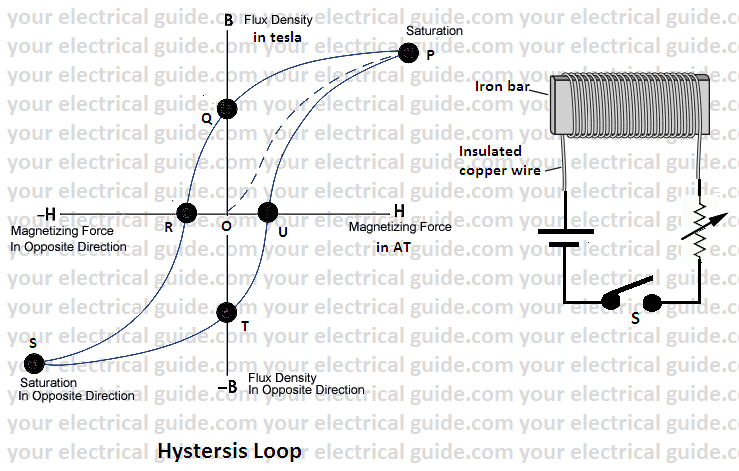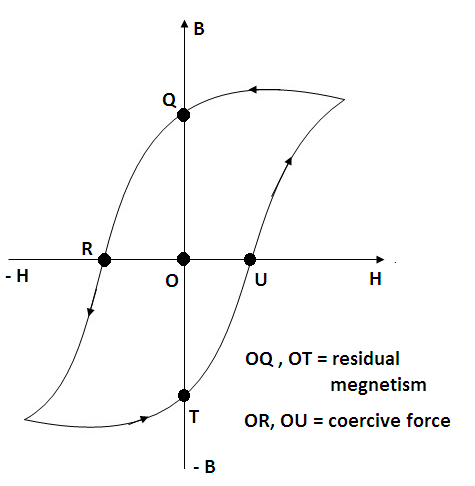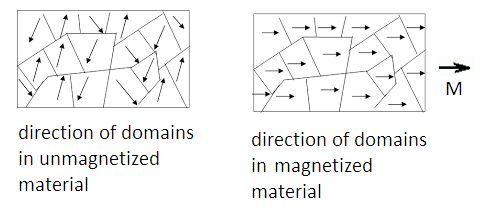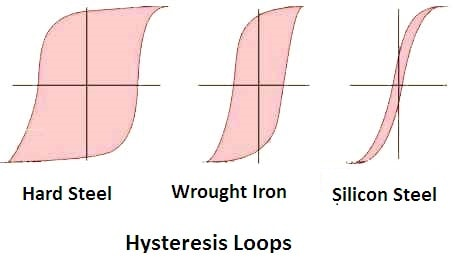# Hysteresis Loop | Loss in Magnetic Material

When a ferromagnetic material is placed in a magnetic field, it is magnetized by induction. If we vary the magnetic intensity H of the magnetic field, the magnetic flux density B in the ferromagnetic material does not vary linearly with H i.e. the susceptibility and the permeability of the material are not constants but vary with H and also depends upon the past history of the material.

# How hysteresis loop is formed?

To form the hysteresis loop of a ferromagnetic material, let us take an unmagnetized bar of iron and magnetize it by placing it within the magnetic field of a solenoid. We will magnetize the bar first in one direction and then in opposite direction by reversing the direction of current through the coil.

The field H produced by the solenoid is called the magnetizing field or force H. It produces magnetic flux density B in the iron bar. The value of H can be increased or decreased by increasing or decreasing current. Now plot values of B for different values of H.• Let us start from point O where H = 0.
• As H is increased further by increasing the current I, B increases and reaches its maximum value at point P. At the point, P iron bar acquires a state of magnetic saturation. Any further increase in H does not produce any increase in B.
• If now the magnetizing field H is decreased, the flux density B also decreases following a new path PQ (not the original path OP).
•

• Thus, B lags behind H. When H becomes zero, B still has the value equal to OQ.
• Now reverse the direction of H by reversing the direction of current I. When H = – OR, B = 0 and the iron bar is demagnetized.
• When H is further increased in the reversed direction, the iron bar is increasingly magnetized in the opposite direction B reaches its maximum value at point S.
• At point S, iron bar is again magnetically saturated.
•

• By taking H back from its maximum negative value (through zero) to its original maximum positive value, a symmetrical curve STUP is obtained
• This time also, when H is decreased to zero, B is not zero and has a value equal to OT.

Thus, it is found the magnetic flux density B always lags behind the magnetizing field H. The lagging of B behind H is called ‘hysteresis’. The closed curve so obtained is known as ‘hysteresis loop’. On repeating the process, the same curve is traced again but the portion OP is never obtained.

# What are retentivity and coercivity?

The magnetization remaining in the material when the magnetizing field is reduced to zero is called the ‘residual magnetism’.The power of retaining residual magnetism is called the ‘retentivity’ of the material.

The residual magnetism of a material can be wiped off by applying magnetizing force in opposite direction. The value of magnetizing force required to wipe of its residual magnetism is called corrosive force.

Thus coercivity of a material is a measure of reverse magnetizing field required to destroy the residual magnetism of the material.

# Hysteresis Loss in Magnetic Material

A ferromagnetic material consists of local regions called ‘domains’, each of which is spontaneously magnetized. In an unmagnetized material the directions of magnetization in different domains are different so that, on the average, the resultant magnetization is zero.When we magnetize the material by placing it in an external magnetizing field, then those domains which are favorably oriented with respect to the external field grow in size, and also each domain rotates so that its direction of magnetization becomes aligned with the field direction.

During this process, energy is taken by the material.

When the external field is removed, the domain boundaries do not move completely back to their original positions, that is, the material retains some magnetization (hysteresis effect). Therefore, the energy supplied to during magnetization is not fully recovered. The balance of energy left in the material lost as heat. This is called hysteresis loss in magnetic material.

Hence, the electrical energy, which is wasted in the form of heat due to the hysteresis of the core material, is known as hysteresis loss in magnetic material.

Hysteresis loss,Ph = ἠBmax1.6 fV watts,

where  Bmax = maximum flux density in Tesla
f = frequency of magnetic reversal in Hz
= NP/120 where N is in r.p.m
V = volume of the core material in m3
ἠ = Steinmetz hysteresis coefficient

This can be proved that the energy lost per unit volume of a material in a complete cycle of magnetization is equal to the area of the hysteresis loop.

This knowledge has a practical application. By observing the hysteresis loop of the ferromagnetic materials, we can get the idea of its magnetic properties. This helps us in selecting the proper material for a particular application. The size and shape of the hysteresis loop of a material depend upon its nature.

## Selection of Magnetic Materials

The choice of a magnetic material for making a permanent magnet, electromagnet, core of a transformer can be decided from the hysteresis loop of the material.Permanent Magnets: The material for a permanent magnet should have high retentivity so that magnet is strong, and high coercivity so that magnetization is not wiped out by stary external fields, mechanical ill-treatment and temperature changes.

The hysteresis loss is immaterial in this case because the material is never put to cyclic changes of magnetization.

It is clear from the hysteresis loop of hard steel; it has high coercivity and retentivity. Therefore it is very suitable for making permanents magnets. Modern permanent magnets are made of alloys ‘ticonal’ and ‘alnico’.

Electromagnets: The material for the cores of electromagnets should have high permeability, especially at low magnetizing fields, and a low retentivity.

The hysteresis loop for wrought iron shows that it has good coercivity and residual magnetism, therefore, it is very suitable for making the core of electromagnets.

Transformer Cores: In this case, the material goes through a complete cycle of magnetization continuously. Therefore, the material must have low hysteresis loss to have less dissipation of energy and hence a small heating of the material (otherwise the insulation of winding may break), a high permeability (to obtain large flux density at the low field) and high specific resistance (to reduce eddy current losses).

Area of the hysteresis loop of silicon steel is very small; therefore it is very suitable for the construction of magnetic parts of electrical machines, which undergo rapid reversal of the magnetic field.

Whereas hard steel has a larger area of the hysteresis loop, therefore there will be a greater hysteresis loss in this material. Hence, hard steel is not suitable for the construction of magnetic parts of electrical machines.

# How hysteresis loss can be reduced?

Hysteresis loss is undesirable in electrical machines as it produces heat and is responsible for the loss of energy thus producing additional temperature rise.

Since hysteresis loss is proportional to the area of the hysteresis loop, the loss is kept low by using materials having narrow hysteresis loops.

Silicon is added to steel for this purpose. For stationary machines like transformers, the silicon content is up to 4% and for rotating electrical machines, it is up to 2%.

Electromagnetism | All Posts

© https://yourelectricalguide.com/ hysteresis loss in magnetic material.